# proof of L'hospitals theorem

13 years ago

Dear sachin,

If f and g are differentiable in a neighborhood of x = c, and f(c) = g(c) = 0, then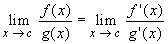provided the limit on the right exists. The same result holds for one-sided limits.

If f and g are differentiable and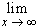f(x) =g(x) = -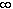then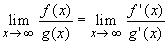provided the last limit exists.

### Proof:

The first part can be proved easily, if the right hand limit equals f'(c) / g'(c): Since f(c) = g(c) = 0 we have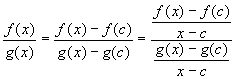Taking the limit as x approaches c we get the first result. However, the actual result is somewhat more general, and we have to be slightly more careful. We will use a version of the Mean Value theorem:

Take any sequence {xn} converging to c from above. All assumptions of the generalized Mean Value theorem are satisfied (check !) on [c, xn]. Therefore, for each n there exists a number cn in the interval (c, xn) such that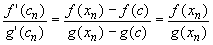All the best.

Sagar Singh

B.Tech IIT Delhi

13 years ago

Consider the linear approximation to f(x) and g(x) at x=a:The ratio of these for x near a is:which, if g'(a) is not 0 approaches f '(a) / g'(a) as x approaches a.

If g'(a) = 0 and f '(a) = 0 we can apply the same rule to the derivatives, to give f "(a) / g"(a).

If these second derivatives are both 0 you can continue to higher derivatives, etc. the result will be the ratio of the first pair of non-vanishing higher derivatives at a.

Of course if the first non-vanishing derivative of the numerator is the kth and occurs before the kth then the ratio is 0; if the first non-vanishing entry of the denominator occurs after that of the numerator, the ratio goes to infinity at a.